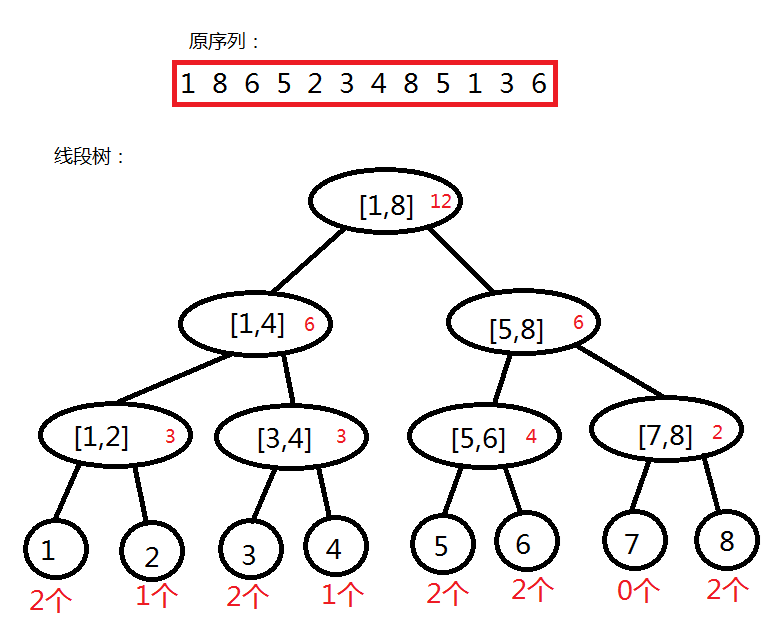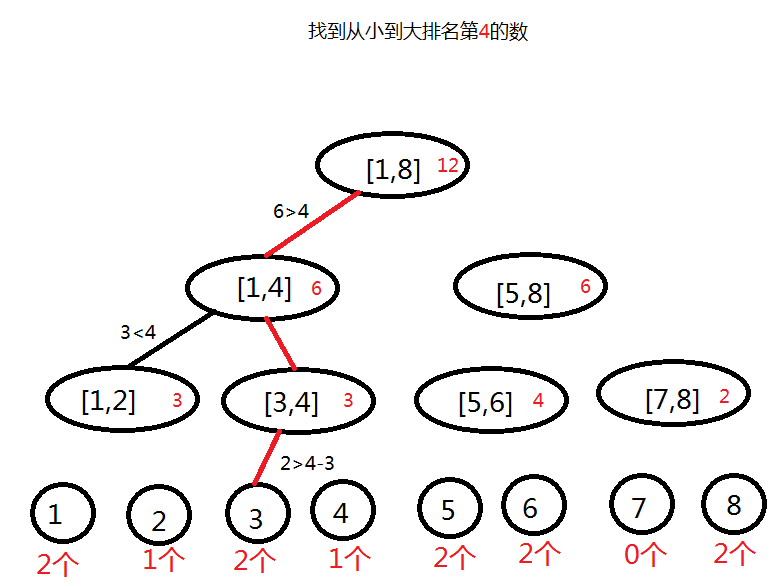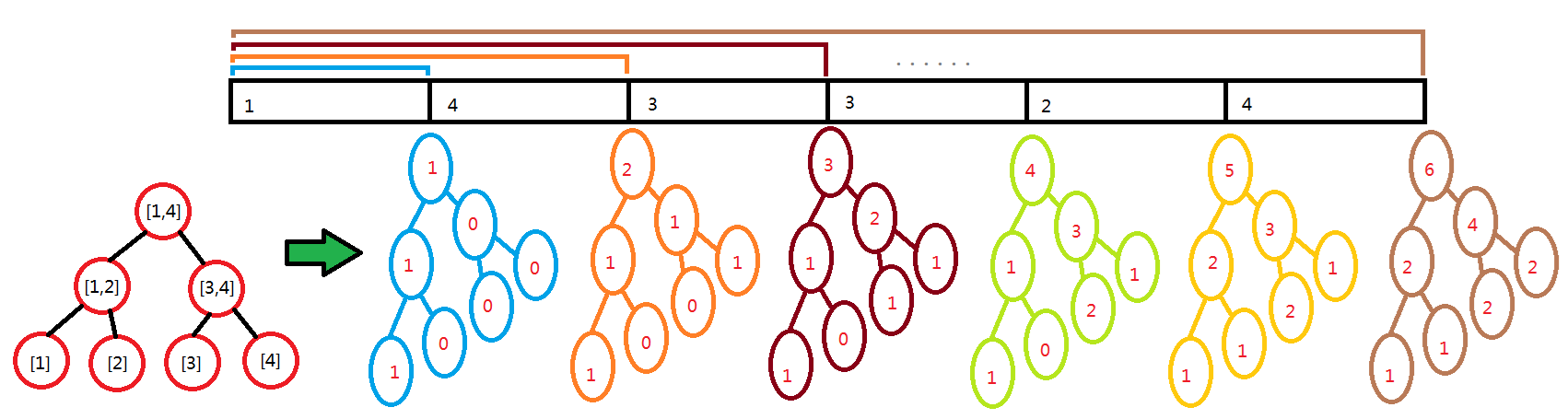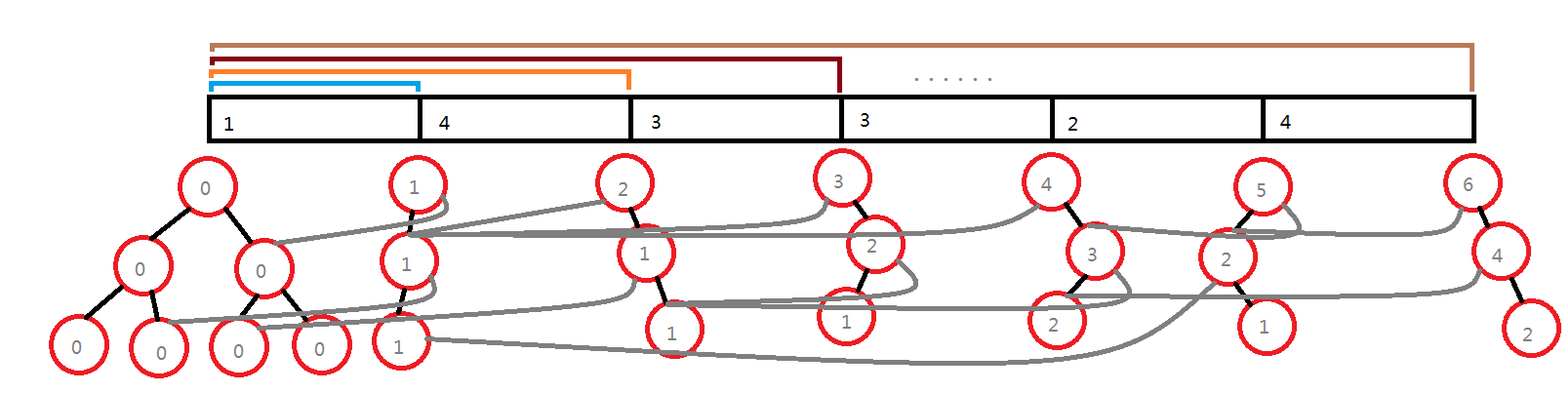• //可持久化权值线段树 const int maxn = 2e5 + 5; struct e{ int l, r, sum = 0; }tree[20 * maxn];//tree存的是所有叶子节点 //现在的树不再是2*i左子树2*i+1右子树了，所有子树都要保存左右节点在tree里的编号 ...

https://www.luogu.com.cn/problem/P3834
解决静态区间第k小的问题。

#include<bits/stdc++.h>
using namespace std;
const int maxn = 2e5 + 5;
int tot, n, m;
int sum[maxn << 5], rt[maxn], ls[maxn << 5], rs[maxn << 5];
int a[maxn], ind[maxn], len;
inline int getid(const int &val)
{
return lower_bound(ind + 1, ind + len + 1, val) - ind;
}
int build(int l, int r)
{
int root = ++tot;
if (l == r) return root;
int mid = l + r >> 1;
ls[root] = build(l, mid);
rs[root] = build(mid + 1, r);
return root;
}
int update(int k, int l, int r, int root)
{
int dir = ++tot;
ls[dir] = ls[root], rs[dir] = rs[root], sum[dir] = sum[root] + 1;
if (l == r) return dir;
int mid = l + r >> 1;
if (k <= mid) ls[dir] = update(k, l, mid, ls[dir]);
else rs[dir] = update(k, mid + 1, r, rs[dir]);
return dir;
}
int query(int u, int v, int l, int r, int k)
{
if (l == r) return l;
int mid = l + r >> 1, x = sum[ls[v]] - sum[ls[u]];
if (k <= x) return query(ls[u], ls[v], l, mid, k);
else return query(rs[u], rs[v], mid + 1, r, k - x);
}
inline void init()
{
memcpy(ind, a, sizeof(a));
sort(ind + 1, ind + n + 1);
len = unique(ind + 1, ind + n + 1) - ind - 1;
rt = build(1, len);
}
int main()
{
scanf("%d %d", &n, &m);
for (int i = 1; i <= n; ++i) scanf("%d", a + i);
init();
for (int i = 1; i <= n; ++i) rt[i] = update(getid(a[i]), 1, len, rt[i - 1]);

int l, r, k;
while (m--) {
scanf("%d %d %d", &l, &r, &k);
printf("%d\n", ind[query(rt[l - 1], rt[r], 1, len, k)]);
}
return 0;
}

My Style

#include<bits/stdc++.h>
using namespace std;
//可持久化权值线段树
const int maxn = 2e5 + 5;
struct e{
int l, r, sum = 0;
}tree[20 * maxn];//tree存的是所有叶子节点
//现在的树不再是2*i左子树2*i+1右子树了，所有子树都要保存左右节点在tree里的编号
struct dis{
int v, id;
}a[maxn];

int tot, root[maxn], rk[maxn];//cnt代表开了多少叶子节点
//那么root代表啥？因为每次插入的时候会从根节点开始新加一条链
//那么就相当于多个root节点了，root[i]存的是第i次插入分裂出来的根在tree里的下标
bool cmp(const dis &x, const dis &y){return x.v < y.v;}
//返回根节点
int update(int l, int r, int rt, int k)//rt代表需要复制的节点下标
{
int dir = ++tot;//新开节点的下标
tree[dir] = tree[rt];//复制前一个根节点
++tree[dir].sum;//数量+1
if (l == r) return dir;//返回当前根节点
int m = (l + r) >> 1;
//如果在左边，那么左边分裂
if (k <= m) tree[dir].l = update(l, m, tree[rt].l, k);
//否则右边分裂
else tree[dir].r = update(m + 1, r, tree[rt].r, k);
return dir;
}
//返回的是那个点的位置
int query(int l, int r, int x, int y, int k)
{
if (l == r) return l;
int num = tree[tree[y].l].sum - tree[tree[x].l].sum;
int m = (l + r) >> 1;
if (k <= num) return query(l, m, tree[x].l, tree[y].l, k);
else return query(m + 1, r, tree[x].r, tree[y].r, k - num);
}
int main()
{
int n, m;
scanf("%d %d", &n, &m);
for (int i = 1; i <= n; ++i) scanf("%d", &a[i].v), a[i].id = i;
sort(a + 1, a + 1 + n, cmp);
for (int i = 1; i <= n; ++i) rk[a[i].id] = i;//每个点的值是全部值第几小的

for (int i = 1; i <= n; ++i){
root[i] = update(1, n, root[i - 1], rk[i]);
}

int l, r, k;
while (m--){
scanf("%d %d %d", &l, &r, &k);
printf("%d\n", a[query(1, n, root[l - 1], root[r], k)].v);
}
return 0;
}

展开全文算法
• 前言很久以前就听说过主席树这个名字，当时听到的时候还只能一脸懵逼，不知道是什么意思。而现在学习了“线段树”之后终于可以对这种神奇的“线段树”进行学习。

前言

很久以前就听说过主席树这个名字，当时听到的时候还只能一脸懵逼，不知道是什么意思。而现在学习了“线段树”之后终于可以对这种神奇的“线段树”进行学习。虽然说我并不知道“主席树”与可持久化权值线段树到底有着什么样的联系，甚至不知道什么是“可持久化”，但是这两个陌生的名词在机房学长们的口中就好像是同一个意思，因此我把这篇文章的标题写成了：“主席树——可持久化权值线段树”。以下是机房学长的两篇博客。

goseqh ‘s 浅谈主席树

高杨大神 ‘s 【模板-7】主席树 Orz

主席树可以理解成“可持久化权值线段树”，由此观之，“线段树”的知识对于“主席树”的学习来说是尤为重要的，没有学过“线段树”的同学建议看一下有关的博客：

我的博客：我与线段树的故事

（有“主席树”为什么没有“总理树”，╮(╯▽╰)╭哎~）

1.线段树的“随波逐流”

线段树是我学习的第一种“牛13”的数据结构，它可以支持强大的区间修改和区间查询等操作。后来我遇到了这样的一道题：给定一个序列，询问这个区间的“区间第k大”。本以为支持强大区间操作的“线段树”能够胜任，结果发现“线段树”捉襟见肘了。我怎么也想不到一种方发用线段树维护出“区间第k大的属性”，因为这个区间属性太特殊了，这怎么办呢？

在学习线段树的时候，我们可以用O（lgn）的时间用二分法查找一个结点所处的位置，这是线段树中结点与原序列的关系。而现在我们要找到的是“第k大”，假如给原序列排序，排序之后的“第k个”就是我想要找的答案。这次，我们要找到的就不是“结点与原序列的关系了”而是“结点与‘数集’之间的关系”。也就是说我们可以为”数集”建立一棵线段树。在这棵线段树里，每一个结点表示一个“数值区间”，结点的属性表示原序列有多少个数值在这个“数值区间”当中，它的建立和其他的线段树也是没什么两样的。如果我统计出了每一个“数值区间”所包含的元素个数就可以很方便的确定“第k大”的数了。假如原序列的数值范围为[1,8]，我要找到它的“第k大”。我就要去判断“数值范围”在[1,4]中的数个数是否大于k。如果是，说明第k大的数在[1,4]，否则在[1,8]，这样就可以递归求出这个序列的“k大值”。2.主席树

你会发现，如果只用刚才的那个“数值区间”的线段树是不能求出“区间第k大”的，因为它只能求出一个序列整体的“第k大”，而不能拓展到任意区间。然而有这样的一个性质：如果我们用“M(i,j,x,y)”表示原序列中 从第i个数开始到第j个数结束 的区间 满足“数值区间”[x,y]的元素的个数，那么就有:

M ( i , j , x , y ) = M ( 1 , j , x , y ) - M ( 1 , i - 1 , x , y )

（看明白之后往下看。）

这就说明，我不用给每个区间建立线段树，只要给原序列的每个前缀建立一个线段树就能求得所有区间与“数集”的关系。也就是说我们可以建立“一群线段树”来维护这个性质：这看起来是不是像一个立体的结构。

不过要注意的是，这里的每一棵线段树都有MlgM个结点，一共有N棵线段树，所以说空间复杂度一定会炸，而且构建这么多完整的线段树时间也一定会炸。但是你会发现，其实这些线段树都是非常相似的，因为两个长度只差1的前缀之间只有一个不同的数，因此它与前一个线段树只有一条支路是不同呢？那么为什么不让这些线段树共用一部分树枝呢？这听起来很疯狂，不过却很可行。我们可以先为“空前缀”建立一棵“空线段树”，里面的权值全是零。然后依次为每一个前缀建立线段树，与前一棵树相同的区间直接接到前一棵树的对应节点上，不同的部分再新申请结点。比如：当我构造当前前缀的线段树的[1,4]数值区间时，如果当前前缀比上一个前缀新增的那个数为3或4，就把当前结点的左子接到上一个线段树的[1,2]区间上；如果新增的那个数为1或2，就把当前结点的右子接到上一个节点的[3,4]区间上。然后再用同样的方法递归处理新添加的结点。这样除了空树以外，每一个前缀只需要lgM个结点用于建树，空间复杂度为O（（N+M）lgM），比较可以接受。

我们可以储存一个treeRoot数组，用treeRoot[i]表示第i个前缀所对应的线段树的根节点，以便于以treeRoot[i+1]为根的线段树的构建。

3.主席树的构造

给出我自己的模板，我的代码可能比较有个性：

#include<iostream>
#include<cstdlib>
using namespace std;

struct NODE//定义树节点
{
int l,r;
int lch,rch;
int num_count;
void clr(int L,int R,int LCH,int RCH, int NUM_COUNT)
{
l=L;r=R;lch=LCH;rch=RCH;num_count=NUM_COUNT;
}
}ns;
int newnode=0;//当前结点个数(用于申请结点)
int array;//原序列
int root_array;//也就是上文说的treeRoot

void build_empty_tree(int root,int l,int r)//建立一棵空树,"数值范围"为[l,r]
{
if(l==r)//如果确定到唯一数值
{
ns[root].clr(l,r,-1,-1,0);
return;
}
int nl=newnode++;
int nr=newnode++;//申请左子和右子
int mid=(l+r)/2;
build_empty_tree(nl,l,mid);
build_empty_tree(nr,mid+1,r);//递归定义左子和右子
ns[root].clr(l,r,nl,nr,0);
}

void build_tree(int last_root,int root_now,int num_now)//建立一个前缀的线段树(局部)
{
ns[root_now]=ns[last_root];//先把原先根节点的内容复制给当前根节点
if(ns[root_now].l==ns[root_now].r)//如果确定到唯一数值
{
ns[root_now].num_count++;//这个数值的统计数加一
return;
}
int mid=(ns[root_now].l+ns[root_now].r)/2;
if(num_now<=mid)//如果新添的数值在左子树
{
ns[root_now].lch=newnode++;//新申请一个左子结点
build_tree(ns[last_root].lch,ns[root_now].lch,num_now);//递归定义左子
}else
if(mid<num_now)//如果在右子树
{
ns[root_now].rch=newnode++;//申请一个右子结点
build_tree(ns[last_root].rch,ns[root_now].rch,num_now);//递归定义右子
}
ns[root_now].num_count++;
}

int query(int root_i,int root_j,int k)//询问两颗线段树之间所夹区间"k大值"
{
if(ns[root_j].l==ns[root_j].r)//确定到唯一结点
return ns[root_j].l;
int left_count=(ns[ns[root_j].lch].num_count)-(ns[ns[root_i].lch].num_count);//左子中数字个数
if(k<=left_count)//k大值在左子
{
int a=query(ns[root_i].lch,ns[root_j].lch,k);
return a;
}else{//k大值在右子
int a=query(ns[root_i].rch,ns[root_j].rch,k-left_count);
return a;
}
}

int main()
{
int n;
cout<<"n=";//输入序列长度
cin>>n;
int x,y;
cout<<"x,y=";//输入数值范围
cin>>x>>y;
cout<<"array[1..n]=";//输入序列
for(int i=1;i<=n;i++)
cin>>array[i];
root_array=newnode++;//申请空树根结点
build_empty_tree(root_array,x,y);//建空树
for(int i=1;i<=n;i++)
{
int root_now=newnode++;//申请一个新结点
root_array[i]=root_now;
build_tree(root_array[i-1],root_now,array[i]);//为当前前缀建立线段树
}
int m;
cout<<"m=";//输入询问次数
cin>>m;
for(int i=1;i<=m;i++)
{
cout<<"L,R,k=";
int L,R,k;//输入询问,原序列中的[L,R]区间的k大值
cin>>L>>R>>k;
cout<<"query="<<query(root_array[L-1],root_array[R],k)<<endl;//计算询问
}
system("pause");
return 0;
}
展开全文数据结构 算法 C++ 线段树
• 可持久线段树查r + 1 到 n + 1之中大于等于k的第一个元素（必然可以使用） 或已经+1e7的元素（必然可以使用即可） 代码： #include using namespace std; typedef long long ll; const int MAXN = 1e5...

题意：

给定一个1-n的排列，两种操作

1. (1,pos),indicating to change the value of apos to apos+10,000,000;
2. (2,r,k),indicating to ask the minimum value which is **not equal** to any ai ( 1≤i≤r ) and **not less ** than k.

查找不等于a - a[r]间任何元素的且大于等于k的最小值

解题思路：

k数据范围为1e5 操作1加了1e7

所以2操作的最大答案就是n + 1，因为1-n排列的任意值+1e7都完全大于n + 1

这样考虑，如果一个数字+1e7了，说明这个值已经不在这个排列之中了，所以可以直接使用作为答案

那么问题分解为查找一个最接近k的元素不等于1-r之中任意元素或r + 1 到 n + 1之间任意元素

那么可以保存一个set记录不在排列中的值

用可持久线段树查r + 1 到 n + 1之中大于等于k的第一个元素（必然可以使用）

或已经+1e7的元素（必然可以使用即可）

代码：

#include <bits/stdc++.h>
using namespace std;
typedef long long ll;
const int MAXN = 1e5 + 100;
int root[MAXN], tot = 0;
struct node
{
int ls, rs, val;
}t[MAXN << 5];

void update(int l, int r, int &now, int lst, int pos)
{
now = ++tot;
t[now] = t[lst];
++t[now].val;
if(l == r)	{ return ; }
int mid = (l + r) >> 1;
if(pos <= mid) update(l, mid, t[now].ls, t[lst].ls, pos);
else update(mid + 1, r, t[now].rs, t[lst].rs, pos);
}

int fp = 0;

void find(int l, int r, int now, int pos)
{
if(r < pos || l >= fp)
return ;
if(l == r)
{
fp = min(fp, l);
return ;
}
int mid = (l + r) >> 1;

if(pos <= mid && t[t[now].ls].val)
find(l, mid, t[now].ls, pos);

if(t[t[now].rs].val)
find(mid + 1, r, t[now].rs, pos);
}

int arr[MAXN] = {0};

void init(int n)
{
memset(root, 0, sizeof(root));
tot = 0;
}

int main()
{
ios::sync_with_stdio(0);
cin.tie(0); cout.tie(0);

int T, n, m;

cin >> T;

while(T--)
{
cin >> n >> m;

for(int i = 1; i <= n; ++i)
cin >> arr[i];

init(n);

update(1, n + 1, root[n + 1], root[n + 2], n + 1);

set <int> ST;

ST.insert(n + 1);

for(int i = n; i >= 1; --i)
update(1, n + 1, root[i], root[i + 1], arr[i]);

int lans = 0;

while(m--)
{
int opt, pos, t1, t2, t3, r, k;
cin >> opt;

if(opt == 1)
{
cin >>t1;
t1 ^= lans;
ST.insert(arr[t1] );
}
else
{
cin >> t2 >> t3;

t2 ^= lans; t3 ^= lans;

//cout << t2 << ' ' << t3 << '\n';

fp = n + 1;
find(1, n + 1, root[t2 + 1], t3);
lans = min(fp, *ST.lower_bound(t3));
cout << lans << '\n';
}
}
}

return 0;
}

展开全文• 2021 ICPC 昆明 M Stone Games(可持久化权值线段树） 当时场上就是看了一眼，感觉是线段树，不过并不会写，现在来补一发 题目大意： 给你一个1e6长度的序列a（a[i]<=1e9），和q（q<=1e5）次询问，每次询问区间...

2021 ICPC 昆明 M Stone Games(可持久化权值线段树）

当时场上就是看了一眼，感觉是线段树，不过并不会写，现在来补一发

题目大意：

给你一个1e6长度的序列a（a[i]<=1e9），和q（q<=1e5）次询问，每次询问区间l,r，问从a[l]到a[r]这个子序列所不能表示的最小正整数（表示是指能表示成序列中的一个数或多个数之和），强制在线。

做法

我们先简化问题：一个长度n的序列，如何求他不能表示的最小值呢？
首先可以看到：
区间：1 1 3 20
1.假如区间里没有出现过1，那必然不能表示的最小正整数是1
2.如果1出现了，还有1个1，那我可以用已经有的这个1和这个1组成2（当然1个2也可以）
3.如果它可以组成1~2了，那现在还有一个3，那我们可以用这个3和前面的（0 ~2）表示出3，4，5来
4.现在5已经可以了，我有最后一个一个20，那我们可以搞出0~5+20也就是20 ~25，这时候我们发现6没有出现，那答案是6

我当然不可能这样一直举到1e9去，那么我们来总结下规律：
1.假如我从1到k都可以表示，那么假如我有另一个数m,那我必然可以用这个m和这些1~k组合成m ~m+k的所有数。
2.我现在可以表示出1~k，那么假如说我从1 ~ k+1所有的数的和为sum,那我考虑能不能表示出1 ~sum:
/-------如果sum<=k，说明什么？
首先k+1这个数没有出现
其次前面的数加起来也只有k或更少
那k+1一定表示不出来，答案是k+1
/-------如果sum>k
那按照上面的逻辑，我们可以表示出1~sum的所有值
为啥呢：
我上一步搞出来一个k（k是上一个sum），那假设我现在有一个数x被加进来
我可以表示出x+0~x+k(这个x是小于等于k+1的，所以一定能被它自身或者之前的表示)，
我们能表示出1~k,还能从一个小于等于k+1的x表示到x+k
所以我们可以表示到k+x了
把k+x作为新的k
那我再加入一个y，我们就也能表示出y+0~y+k
所以我能表示出k+x+y了
所以，既然我加进来的都小于k
加多少我就能表示到k+多少
因为一共加了sum-k
所以，我们能表示出sum-k+k=sum了

然后把k变成sum，继续重复这一串步骤

所以，我们每次操作需要取出所有区间内1-k的和
那么我们可以使用可持久化权值线段树来解决

具体操作再说吧，回头搞一篇线段树总结

复杂度

应该是q（询问数） * log（线段树查询） * 奇怪的sum增大过程
这个增长应该是斐波那契起步的，应该是log级别吧（反正我也不太会）

代码

#include<bits/stdc++.h>
using namespace std;
#define ll long long
struct tree{
ll val;
int lc,rc;
};tree t;
int rt,a;
int n,q,cnt=0;
ll maxv=1e9+7;
void build(int l,int r,int pos,int val,int &x,int y){
x=++cnt;
t[x].lc=t[y].lc;
t[x].rc=t[y].rc;
t[x].val=t[y].val+val;
if(l==r) return;
int mid=(l+r)/2;
if(pos<=mid) build(l,mid,pos,val,t[x].lc,t[y].lc);
else build(mid+1,r,pos,val,t[x].rc,t[y].rc);
}
ll query(int l,int r,int ql,int qr,int x,int y){
if(l>=ql&&r<=qr){
return t[y].val-t[x].val;
}
int mid=(l+r)/2;
ll ret=0;
if(ql<=mid) ret+=query(l,mid,ql,qr,t[x].lc,t[y].lc);
if(qr>mid) ret+=query(mid+1,r,ql,qr,t[x].rc,t[y].rc);
return ret;
}
int main(){
cin>>n>>q;
for(int i=1;i<=n;i++){
scanf("%d",&a[i]);
}
for(int i=1;i<=n;i++){
build(1,maxv,a[i],a[i],rt[i],rt[i-1]);
}
ll ans=0;
while(q--){
int l,r;
scanf("%d%d",&l,&r);
l=(l+ans)%n+1;
r=(r+ans)%n+1;
if(l>r) swap(l,r);
ll x=0;
while(1){
ll now=query(1,maxv,1,min(x+1,maxv),rt[l-1],rt[r]);
if(now==x) break;
x=now;
}
ans=x+1;
printf("%lld\n",ans);
}
}

展开全文• 权值线段树可以在[1,n]区间内做Rank操作，但是题目要求在[L,R]内做Rank操作。我们可以想到主席树这种数据结构。解决这道题需要三个函数。 build函数 在一开始的时候要建立一棵空的主席树，即所有叶子节点信息都是0...数据结构 算法
• 求区间x,y内的h-index（最大的h有h...在上x,y区间内二分答案check #include <bits/stdc++.h> typedef long long ll; using namespace std; const int MAXN = 2e5 + 10; int fst, lst; int N; str...
• 他这个增长的话不会低于斐波那契的增长速度，就自己脑补一下吧，所以说咱们可以建立一棵权值线段树，来代表某个权值区间的和是多少，这个复杂度的话应该是n(log*log)，对于1e6的复杂度完全可以，这个题目的时间限制...算法
• [ l , r ]且不属于集合A中的数任选一个数k 所以就需要我们用一个权值可持久化线段树，依次扩大我们当前所能表示的数的范围，直至得到答案。 下面是代码： #include #include #include #include #include #include ...c语言 算法 开发语言
• 通过可持久化建n棵权值线段树，因为最小未出现自然数一定小于数列长度所以权值线段树长度为2e5+1即可,储存每个数最后出现的位置，每次查询l,r,即查询第r棵线段树第一个出现位置小于l的数即可，为了查询还需要保存...
• 主席树经典应用，这一题我们可以对 a 建立权值线段树，每个线段树中每个区间维护的是在这个区间中已经出现的数字的数量。 每插入第 i 个数字我们就建立一个编号为 i 的新的历史版本，这样我们对于一次，一次查询操作...数据结构
• 可持久化权值线段树(主席树) Stone Games | 2020 ICPC昆明站 M题 题意 有一个长度为 NNN 的序列 A[N]A[N]A[N] 有 QQQ 个询问，每次问你 [Li,Ri][L_i,R_i][Li​,Ri​] 中，最小不能被表示的数字 xxx 表示：从这个...算法 数据结构
• 主席树区间求第k大的思路类似权值线段树树求[1,n]第k大。 代码： #include <bits/stdc++.h> using namespace std; const int maxn = 1e5+100; int n,m,tot,rt[maxn]; int a[maxn],b[maxn],len; //离散 ...数据结构 c++ icpc
• 这题的询问是对区间的,因此用区间权值线段树就行了,即可持久化权值线段树. 算法总复杂度O(n*log*log) ps: 也可以直接在树上跑,可以优化掉一个log. code： #include #define ll long long using namespace std; ...
• //与一般权值线段树不同，维护前缀和 if(l==r) return now; ll mid = l+r>>1; if(pos) t[now].ls = update(l,mid,t[rt].ls,pos,val); else t[now].rs = update(mid+1,r,t[rt].rs,pos,val); return now; } ll query...icpc 数据结构
• 静态（非带修）主席树模板（可持久化权值线段树） 洛谷上的主席树模板题 写完后可以自己去交一下，数据已经优化过，必须用主席树写。 接下来是我自己的模板 #include<bits/stdc++.h> using namespace std; ...算法
• 主席模板题，求n个数中，第l个数到第r个数的第ｋ小的数 #include<bits/stdc++.h> using namespace std; #define mid ((l+r)>>1) struct node { int data,l,r; } tree[210000<<5];//主席...
• } //可持久化线段树部分 int a[N*50], b[N*50], root[N*50], tot, len; int lc[N*50] , rc[N*50] , siz[N*50]; void build(int& rt, int l , int r) //采用引用，同时给左右儿子编号，&rt相当于lc[rt],rt变换，lc......